# HashMap 线程安全问题

### 正文 - 准备工作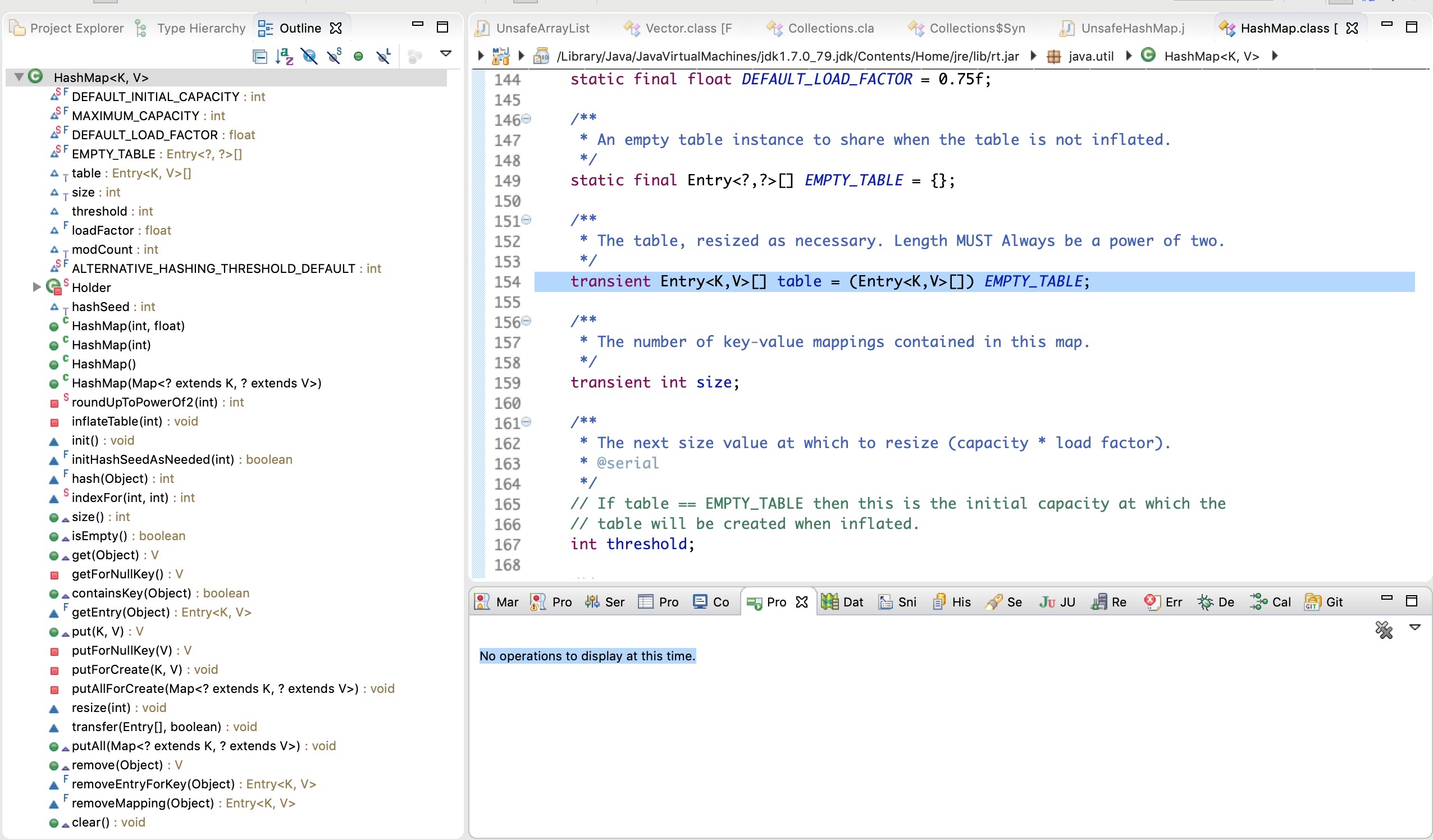transient Entry<K,V>[] table = (Entry<K,V>[]) EMPTY_TABLE;,其中transient是用于表示不需要序列化的标示,我们不用管他.

 static class Entry<K,V> implements Map.Entry<K,V> {
final K key;
V value;
Entry<K,V> next;
int hash;
}


JDK1.7中的HashMap结构大致如下: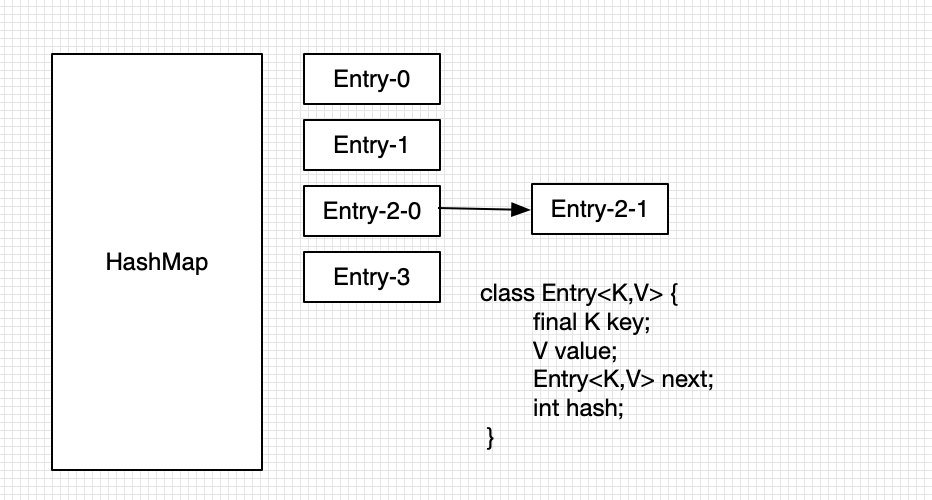### 单线程resize操作

public V put(K key, V value) {
if (table == EMPTY_TABLE) {
// 如果table为空的table,我们需要对其进行初始化操作.
inflateTable(threshold);
}
if (key == null)
// 如果key为null的话 我们对其进行特殊操作(其实是放在table)
return putForNullKey(value);
// 计算hash值
int hash = hash(key);
// 根据hash值获取其在table数组内的位置.
int i = indexFor(hash, table.length);
// 遍历循环链表(结构图类似上图)
for (Entry<K,V> e = table[i]; e != null; e = e.next) {
Object k;
// 如果找到其值的话,将原来的值进行覆盖(满足Map数据类型的特性.)
if (e.hash == hash && ((k = e.key) == key || key.equals(k))) {
V oldValue = e.value;
e.value = value;
e.recordAccess(this);
return oldValue;
}
}
// 如果都没有找到相同都key的值, 那么这个值是一个新值.(直接进行插入操作.)
modCount++;
addEntry(hash, key, value, i);
return null;
}


    void addEntry(int hash, K key, V value, int bucketIndex) {
if ((size >= threshold) && (null != table[bucketIndex])) {
// 如果其大小超过threshold 并且table[index]的位置不为空
// 扩充HashMap的数据结果为原来的两倍
resize(2 * table.length);
hash = (null != key) ? hash(key) : 0;
bucketIndex = indexFor(hash, table.length);
}

createEntry(hash, key, value, bucketIndex);
}


PS: mountCount/size/threshold的联系与区别?

    void resize(int newCapacity) {
Entry[] oldTable = table;
int oldCapacity = oldTable.length;
if (oldCapacity == MAXIMUM_CAPACITY) {
//capacity 容量
threshold = Integer.MAX_VALUE;
return;
}
// 创建一个新的对象
Entry[] newTable = new Entry[newCapacity];
// 将旧的对象内的值赋值到新的对象中
transfer(newTable, initHashSeedAsNeeded(newCapacity));
table = newTable;
// 重新赋值新的临界点
threshold = (int)Math.min(newCapacity * loadFactor, MAXIMUM_CAPACITY + 1);
}

void transfer(Entry[] newTable, boolean rehash) {
// 新table的长度
int newCapacity = newTable.length;
for (Entry<K,V> e : table) {
while(null != e) {
Entry<K,V> next = e.next;
if (rehash) {
e.hash = null == e.key ? 0 : hash(e.key);
}
int i = indexFor(e.hash, newCapacity);
e.next = newTable[i];
newTable[i] = e;
e = next;
}
}
}


1. for (Entry<K,V> e : table) {}外层循环,循环遍历旧table的每一个值.这不难理解,因为要将旧table内的值拷贝进新table.
2. 链表拷贝操作.主要是四行代码.e.next = newTable[i]; newTable[i] = e; e = next;这个操作一下子比较难懂,我们画图了解一下.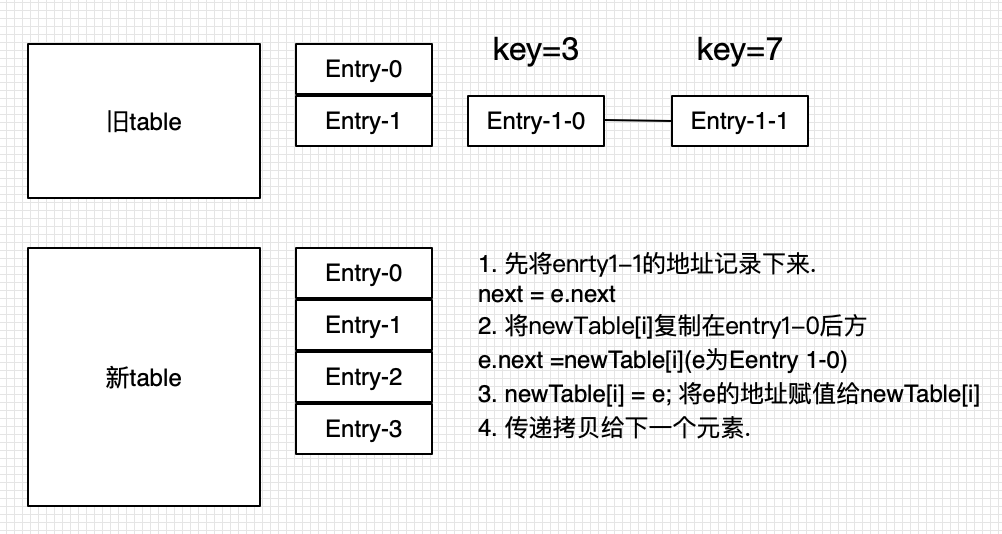这个图还是有点抽象 我们再将细节进行细分.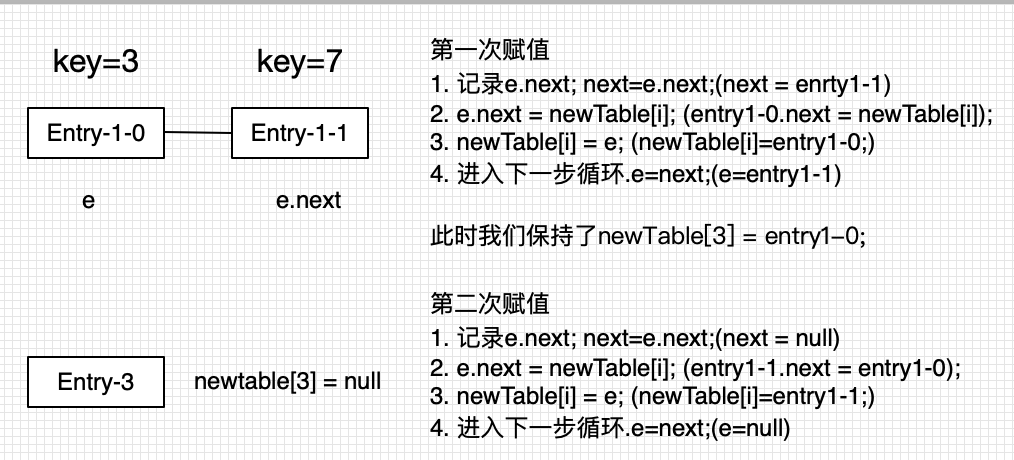1 next = e.next,目的是记录下原始的e.next的地址.图上为Entry-1-1.
2 e.next = newTable[i],也就是将newTable替代了原来的entry1-1.目的是为了记录原来的newTable的链表头.
3 newTable[i] = e,也就死将entry1-0替换成新的链表头.
4. e = next;,循环遍历指针.将e = entry1-1.开始处理entry1-1.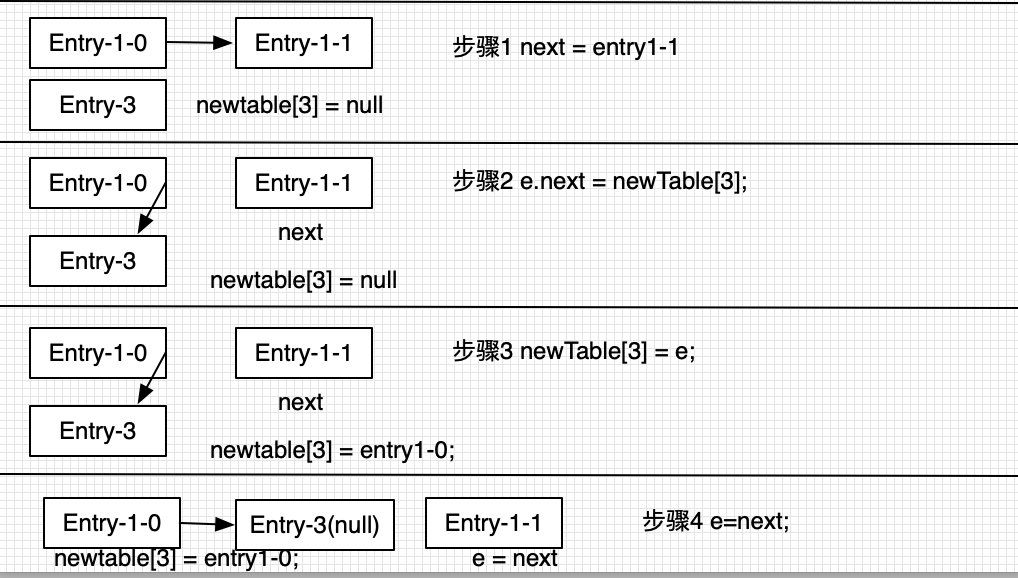(比如 1-0 -> 1-1 ->1-2 插入后将变为 1-2 -> 1-1 -> 1-0)

### 多线程操作 - 分析线程安全?线程非安全?

• Entry<K,V> next = e.next;
• e.next = newTable[i];
• newTable[i] = e;
• e = next;

• 初始(A阻塞 B运行完毕)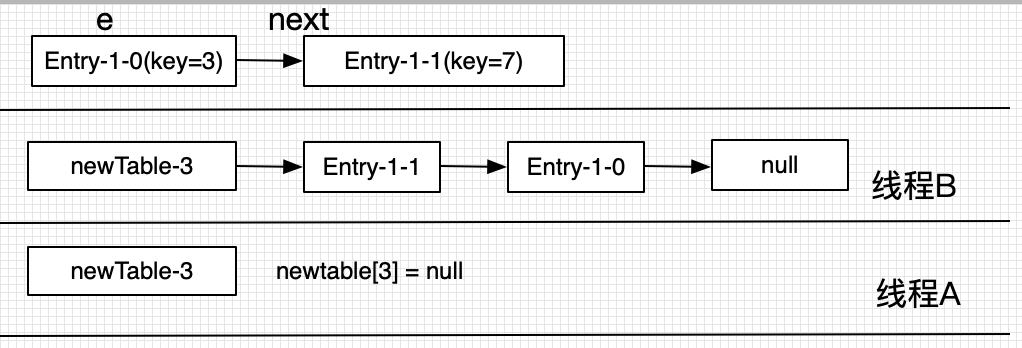• A唤醒 e=key(3)
A的执行步骤如下:
• 线程阻塞前记录 next = key(7)
• 线程唤醒后执行 newTable=null; key(3).next=null;
• newTable=key(3);
• e=next; 即 e = key(7);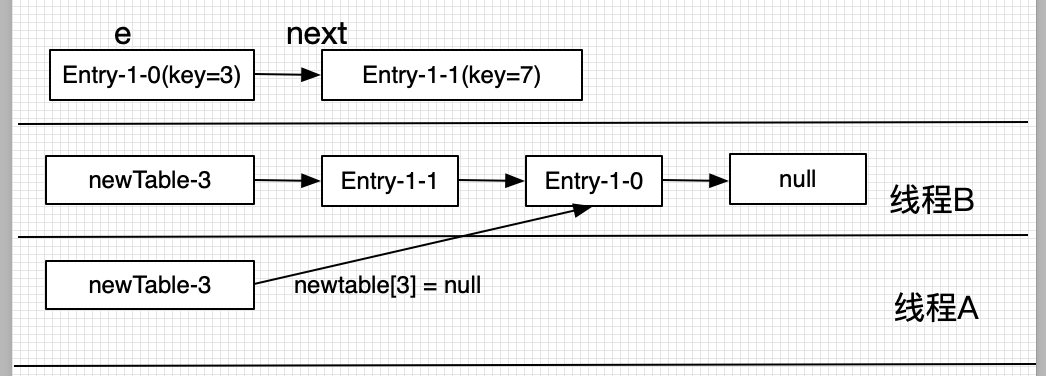• A唤醒 e=key(7)
• next = key(3)
• newTable=key(3); key(7).next=key(3);
• newTable=key(7);
• e=next; 即 e = key(3);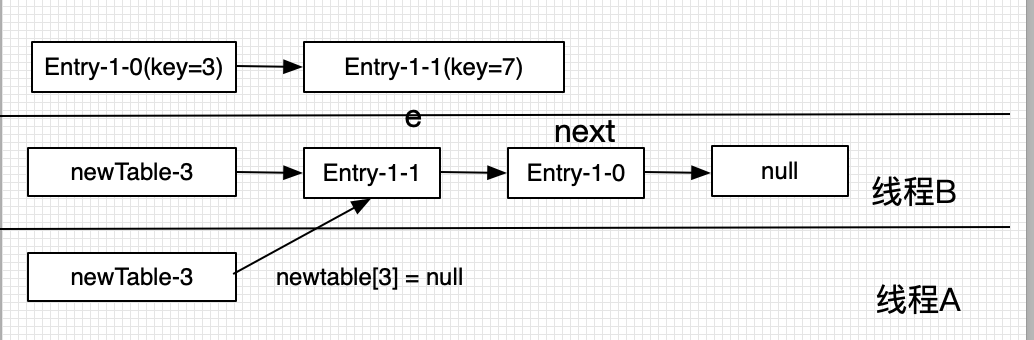• A唤醒 e=key(3)
• next = key(3).next; 即 next = null;
• newTable=key(7); key(3).next=key(7);
• newTable=key(3);
• e=next; 即 e = null; 循环结束.但是此时已经形成了环路.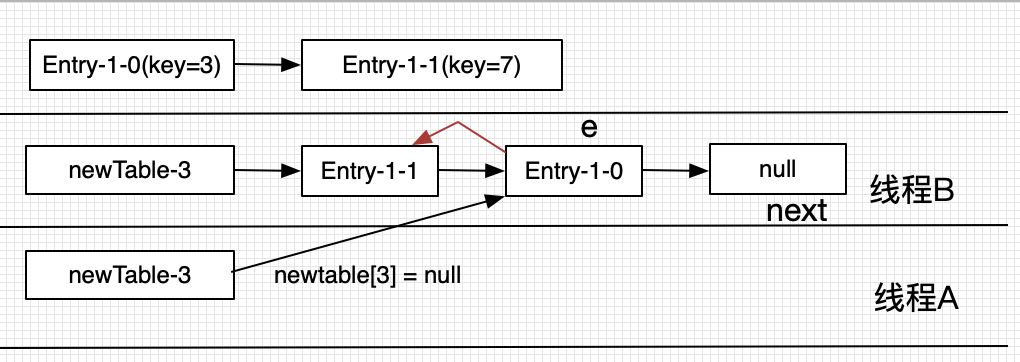Tips

size、capacity、threshold与loadFactor?

• size为hashMap内的链表结点数目.即Entry对象的个数(包括链表内).
• capacity为桶的数目.(即Enrty<k,v> []table的长度) static final int DEFAULT_INITIAL_CAPACITY = 1 << 4; // aka 16
• loadFactor 过载因子static final float DEFAULT_LOAD_FACTOR = 0.75f;
• threshold 边界值.高于边界值,则HashMap进行resize操作.The next size value at which to resize (capacity * load factor). 定义int threshold;

### Reference07-186万+
03-255893
08-041305
03-303万+
06-015386
05-061万+
06-234138
05-111208
11-072954
11-285万+
05-252万+
02-238802
02-284703
02-16459
04-068447
03-31170
05-227万+
09-28389
03-256340
©️2020 CSDN 皮肤主题: Age of Ai 设计师:meimeiellie